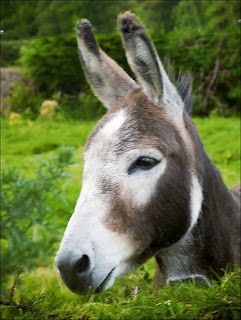## Monday, June 22, 2009

### Math DonkeysI love to give this problem to students.
Two Donkeys are walking down the road loaded down with sacks of potatoes. One looks over at the other and says, "hey, if you give me a sack, I'll have twice as many as you; and if I give you one we'll have the same number. Weird, huh?" The other donkey just says, "Geek" and keeps walking. How many potato sacks does each donkey have?
pic by kaysareKen Colangelo said...

The first donkey has 7 and the other has 5 sacks of potatoes.

If y is the number of sacks on the first donkey and x the second then this is true...
y+1=(x-1)*2
and
y-1=x+1

that reduces to:
y=(2*(x-1))-1
and
y=x+2

We solve for x:
x+2=(2*(x-1))-1
x=(2*(x-1))-3
x=2x-2-3
-x=-2-3
x=5
so, if y=x+2, then y=7

Kate said...

I thought for sure that the second donkey was going to say, "Holy crap! A talking donkey!"

Tinyc Tim said...

d1 = donkey 1's sack count.
d2 = donkey 2's sack count.

(1) d1+1=2(d2-1)
(2) d1-1=d2+1
(3) d1=d2+2 (from (2))
(4) d2+3=2d2-2 (from (1) and (3))
(5) d2=5 (from 4)
(6) d1=7 (from (3) and (5))

So, one of the donkeys has 7 sacks and the other has 5.

vzjp said...

logical deduction and iteration:

Condition 1, implies the two donkeys have an odd number of sacks each.
Condition 2, implies the talker has two more sacks.
So, which two adjacent odd numbers fit the bill?.
3 and 1, no
5 and 3, no
7 and 5, bingo